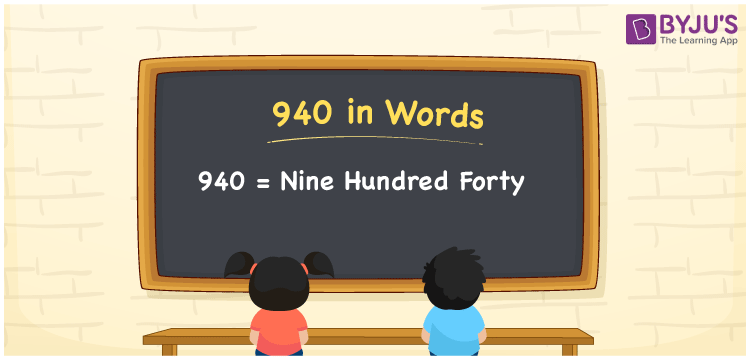# 940 in Words

The number 940 in words can be expressed as Nine Hundred Forty. For example, if you bought a clock watch worth Rs. 940, then you can write, “I bought a clock watch worth Rupees Nine Hundred Forty”. We know that 940 is a cardinal number as it represents a specific quantity or value. Learn the conversion of the number 940 in an easy manner with the help of a place value chart in this article.

 940 in Words Nine Hundred Forty Nine Hundred Forty in numerical form 940

## 940 in English Words

In Mathematics, students are taught to write the numbers in words using the English alphabet. Hence, the number 940 in English words can be expressed as Nine Hundred Forty.## How to Write 940 in Words?

The number 940 can be written in word form using a place value chart. The place value chart helps to determine the position of each digit in the given number. The following table shows the place value chart for the number 940.

 Hundreds Tens Ones 9 4 0

Therefore, we can write the expanded form as:

9 x Hundred + 4 x Ten + 0 x One

= 9 x 100 + 4 x 10 + 0 x 1

= 900 + 40 + 0

= 900 + 40

= 940

= Nine Hundred Forty

Hence, 940 in words is written as Nine Hundred Forty

Interesting way of writing 940 in words

9 = Nine

94 = Ninety-Four

940 = Nine Hundred Forty

Thus, the word form of the number 940 is Nine Hundred Forty

940 is a natural number that precedes 941 and succeeds 939

• 940 in words – Nine Hundred Forty
• Is 940 an odd number? – No
• Is 940 an even number? – Yes
• Is 940 a perfect square number? – No
• Is 940 a perfect cube number? – No
• Is 940 a prime number? – No
• Is 940 a composite number? – Yes

## Frequently Asked Questions on 940 in Words

### Write 940 in words.

We can write 940 in words as Nine Hundred Forty.

### What is the place value of 9 in 940?

The place value of 9 in 940 is Hundreds.

### 940 is a composite number. True or False.

True, the number 940 is a composite number.